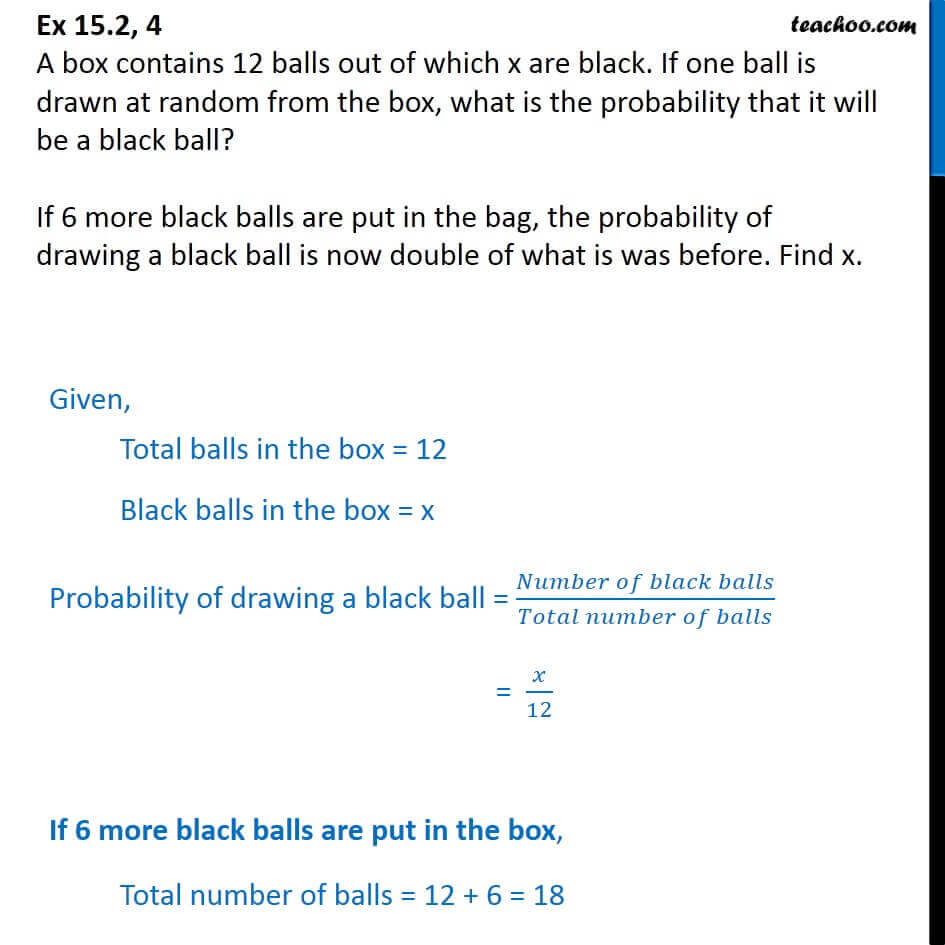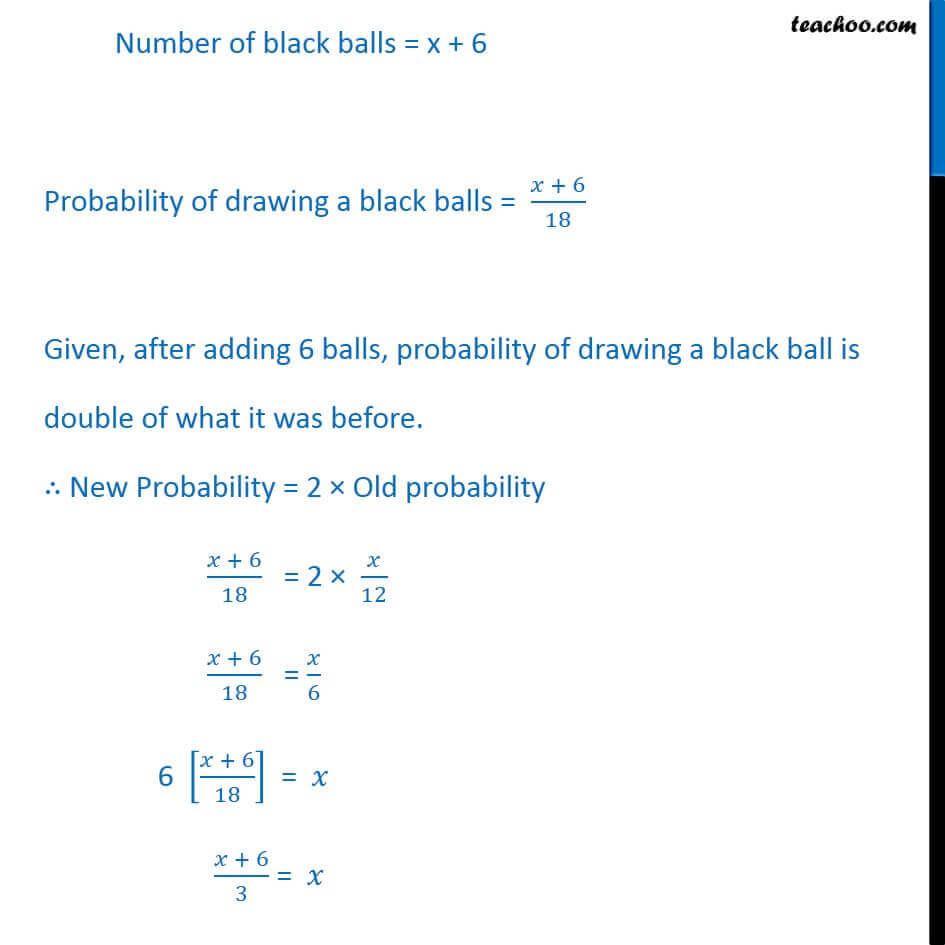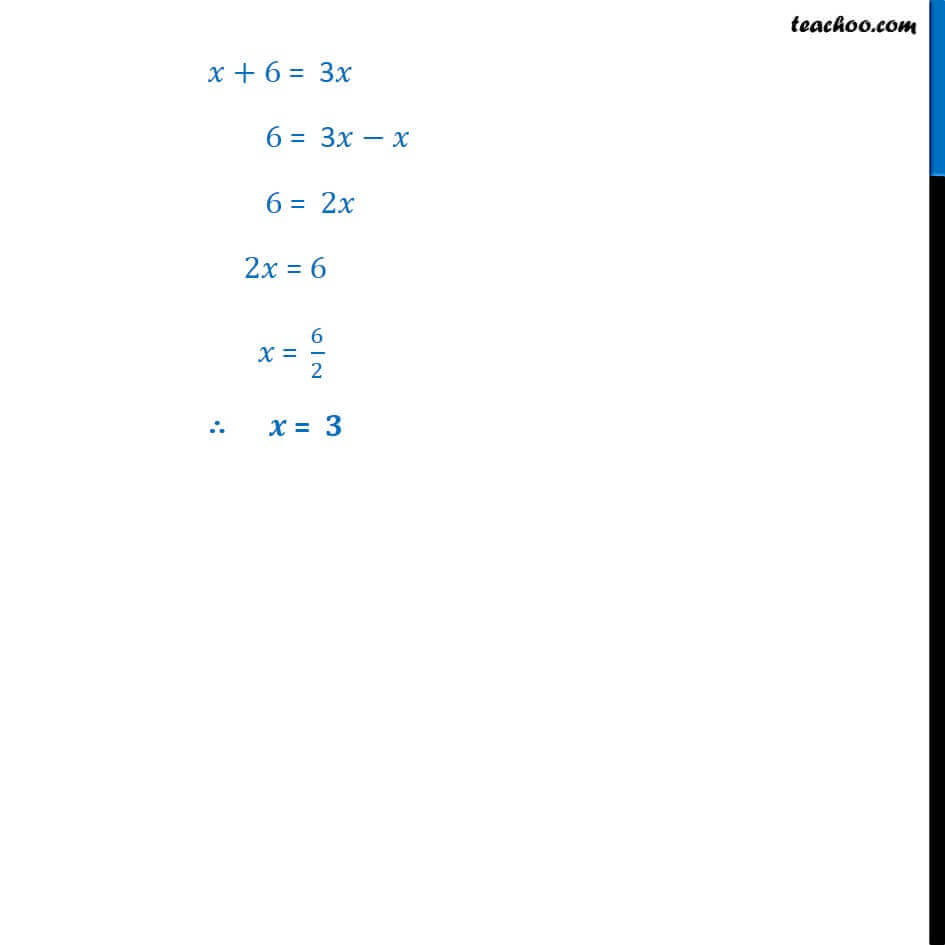Important Probability Questions

Chapter 14 Class 10 Probability
Serial order wiseLearn in your speed, with individual attention - Teachoo Maths 1-on-1 Class

### Transcript

Question 4 A box contains 12 balls out of which x are black. If one ball is drawn at random from the box, what is the probability that it will be a black ball? If 6 more black balls are put in the bag, the probability of drawing a black ball is now double of what is was before. Find x. Given, Total balls in the box = 12 Black balls in the box = x Probability of drawing a black ball = (𝑁𝑢𝑚𝑏𝑒𝑟 𝑜𝑓 𝑏𝑙𝑎𝑐𝑘 𝑏𝑎𝑙𝑙𝑠)/(𝑇𝑜𝑡𝑎𝑙 𝑛𝑢𝑚𝑏𝑒𝑟 𝑜𝑓 𝑏𝑎𝑙𝑙𝑠) = 𝑥/12 If 6 more black balls are put in the box, Total number of balls = 12 + 6 = 18 Number of black balls = x + 6 Probability of drawing a black balls = (𝑥 + 6)/18 Given, after adding 6 balls, probability of drawing a black ball is double of what it was before. ∴ New Probability = 2 × Old probability (𝑥 + 6)/18 = 2 × 𝑥/12 (𝑥 + 6)/18 = 𝑥/6 6 [(𝑥 + 6)/18] = 𝑥 (𝑥 + 6)/3 = 𝑥 𝑥+6 = 3𝑥 6 = 3𝑥−𝑥 6 = 2𝑥 2𝑥 = 6 𝑥" = " 6/2 ∴ 𝒙 = 𝟑